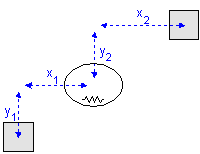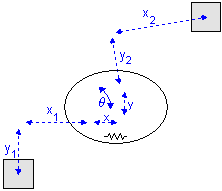﻿ 20-sim webhelp > Library > Iconic Diagrams > Mechanical > Translation > 2DSmallRotation > TwoDSpring

# TwoDSpring

Navigation:  Library > Iconic Diagrams > Mechanical > Translation > 2DSmallRotation >

# TwoDSpring## Library

Iconic Diagrams\Mechanical\Translation\2DSmallAngles

## Use

Domains: Continuous. Size: 2-D. Kind: Iconic Diagrams (2D Planar).

## Description

A TwoDSpring model represents a flexible connection between two bodies. The bodies are located relative to the center of stiffness. The offsets from the bodies to the center of stiffness are indicated by x1, y1, x2 and y2 as shown in the picture below (left).A 2-D spring in rest (left) and stretched (right).

Note that x1, y1, x2 and y2 are defined with respect to the spring coordinate frame which is at the center of the spring. This means in the picture above x1 and y1 have a negative value and x2 and y2 have a positive value.

When the spring is stretched the offsets will change by the spring elongations x, y and q as indicated in the picture (right). The center of stiffness is always located precisely in the middle of the spring elongations. Because all spring equations will be calculated from the center of stiffness this means that the offsets for a stretched spring are (x1 - x/2), (y1 - y/2), (x2 + x/2) and (y2 + y/2).

For a realistic representation of vibration behavior, every 2D spring has a damper in parallel. If you want to turn off the effects of this damper, choose the damping parameters equal to zero. If you want to damp out unwanted vibrations, choose the damping parameters equal to 0.1% to 10% of the spring parameters.

## Interface

 Ports Description P Port with three degrees of freedom (x, y, q). Causality fixed force out P Parameters x1 distance in x-direction from center of stiffness to port 1 [m] y1 distance in y-direction from center of stiffness to port 1 [m] x2 distance in x-direction from center of stiffness to port 2 [m] y2 distance in y-direction from center of stiffness to port 2 [m] kx stiffness in x-direction [N/m] ky stiffness in y-direction [N/m] kth rotational stiffness [N.m/rad] dx damping in x-direction [N.s/m] dy damping in y-direction [N.s/m] dth rotational damping [N.m.s/rad] Initial Values x_initial The initial extension of the spring [m]. y_initial The initial extension of the spring [m]. theta_initial The initial rotation of the spring [rad].